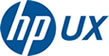BN_mod man page on HP-UX

 Man page or keyword search:   man All Sections 1 - General Commands 2 - System Calls 3 - Subroutines, Functions 4 - Special Files 5 - File Formats 6 - Games and Screensavers 7 - Macros and Conventions 8 - Maintenence Commands 9 - Kernel Interface New Commands Server 4.4BSD AIX Alpinelinux Archlinux Aros BSDOS BSDi Bifrost CentOS Cygwin Darwin Debian DigitalUNIX DragonFly ElementaryOS Fedora FreeBSD Gentoo GhostBSD HP-UX Haiku Hurd IRIX Inferno JazzOS Kali Knoppix LinuxMint MacOSX Mageia Mandriva Manjaro Minix MirBSD NeXTSTEP NetBSD OPENSTEP OSF1 OpenBSD OpenDarwin OpenIndiana OpenMandriva OpenServer OpenSuSE OpenVMS Oracle PC-BSD Peanut Pidora Plan9 QNX Raspbian RedHat Scientific Slackware SmartOS Solaris SuSE SunOS Syllable Tru64 UNIXv7 Ubuntu Ultrix UnixWare Xenix YellowDog aLinux   10987 pages apropos Keyword Search (all sections) Output format html ascii pdf view pdf save postscript[printable version]

```BN_add(3)			    OpenSSL			     BN_add(3)

NAME
BN_mod_sub, BN_mod_mul, BN_mod_sqr, BN_exp, BN_mod_exp, BN_gcd - arith‐
metic operations on BIGNUMs

SYNOPSIS
#include <openssl/bn.h>

int BN_add(BIGNUM *r, const BIGNUM *a, const BIGNUM *b);

int BN_sub(BIGNUM *r, const BIGNUM *a, const BIGNUM *b);

int BN_mul(BIGNUM *r, BIGNUM *a, BIGNUM *b, BN_CTX *ctx);

int BN_sqr(BIGNUM *r, BIGNUM *a, BN_CTX *ctx);

int BN_div(BIGNUM *dv, BIGNUM *rem, const BIGNUM *a, const BIGNUM *d,
BN_CTX *ctx);

int BN_mod(BIGNUM *rem, const BIGNUM *a, const BIGNUM *m, BN_CTX *ctx);

int BN_nnmod(BIGNUM *r, const BIGNUM *a, const BIGNUM *m, BN_CTX *ctx);

int BN_mod_add(BIGNUM *r, BIGNUM *a, BIGNUM *b, const BIGNUM *m,
BN_CTX *ctx);

int BN_mod_sub(BIGNUM *r, BIGNUM *a, BIGNUM *b, const BIGNUM *m,
BN_CTX *ctx);

int BN_mod_mul(BIGNUM *r, BIGNUM *a, BIGNUM *b, const BIGNUM *m,
BN_CTX *ctx);

int BN_mod_sqr(BIGNUM *r, BIGNUM *a, const BIGNUM *m, BN_CTX *ctx);

int BN_exp(BIGNUM *r, BIGNUM *a, BIGNUM *p, BN_CTX *ctx);

int BN_mod_exp(BIGNUM *r, BIGNUM *a, const BIGNUM *p,
const BIGNUM *m, BN_CTX *ctx);

int BN_gcd(BIGNUM *r, BIGNUM *a, BIGNUM *b, BN_CTX *ctx);

DESCRIPTION
BN_add() adds a and b and places the result in r ("r=a+b").  r may be
the same BIGNUM as a or b.

BN_sub() subtracts b from a and places the result in r ("r=a-b").

BN_mul() multiplies a and b and places the result in r ("r=a*b").  r
may be the same BIGNUM as a or b.  For multiplication by powers of 2,
use BN_lshift(3).

BN_sqr() takes the square of a and places the result in r ("r=a^2"). r
and a may be the same BIGNUM.  This function is faster than
BN_mul(r,a,a).

BN_div() divides a by d and places the result in dv and the remainder
in rem ("dv=a/d, rem=a%d"). Either of dv and rem may be NULL, in which
case the respective value is not returned.  The result is rounded
towards zero; thus if a is negative, the remainder will be zero or neg‐
ative.  For division by powers of 2, use BN_rshift(3).

BN_mod() corresponds to BN_div() with dv set to NULL.

BN_nnmod() reduces a modulo m and places the non-negative remainder in
r.

BN_mod_add() adds a to b modulo m and places the non-negative result in
r.

BN_mod_sub() subtracts b from a modulo m and places the non-negative
result in r.

BN_mod_mul() multiplies a by b and finds the non-negative remainder
respective to modulus m ("r=(a*b) mod m"). r may be the same BIGNUM as
a or b. For more efficient algorithms for repeated computations using
the same modulus, see BN_mod_mul_montgomery(3) and BN_mod_mul_recipro‐
cal(3).

BN_mod_sqr() takes the square of a modulo m and places the result in r.

BN_exp() raises a to the p-th power and places the result in r
("r=a^p"). This function is faster than repeated applications of
BN_mul().

BN_mod_exp() computes a to the p-th power modulo m ("r=a^p % m"). This
function uses less time and space than BN_exp().

BN_gcd() computes the greatest common divisor of a and b and places the
result in r. r may be the same BIGNUM as a or b.

For all functions, ctx is a previously allocated BN_CTX used for tempo‐
rary variables; see BN_CTX_new(3).

Unless noted otherwise, the result BIGNUM must be different from the
arguments.

RETURN VALUES
For all functions, 1 is returned for success, 0 on error. The return
value should always be checked (e.g., "if (!BN_add(r,a,b)) goto err;").
The error codes can be obtained by ERR_get_error(3).

HISTORY
BN_add(), BN_sub(), BN_sqr(), BN_div(), BN_mod(), BN_mod_mul(),
BN_mod_exp() and BN_gcd() are available in all versions of SSLeay and
OpenSSL. The ctx argument to BN_mul() was added in SSLeay 0.9.1b.
BN_exp() appeared in SSLeay 0.9.0.  BN_nnmod(), BN_mod_add(),
BN_mod_sub(), and BN_mod_sqr() were added in OpenSSL 0.9.7.

```
[top]

List of man pages available for HP-UX

Copyright (c) for man pages and the logo by the respective OS vendor.

For those who want to learn more, the polarhome community provides shell access and support.

 Vote for polarhome